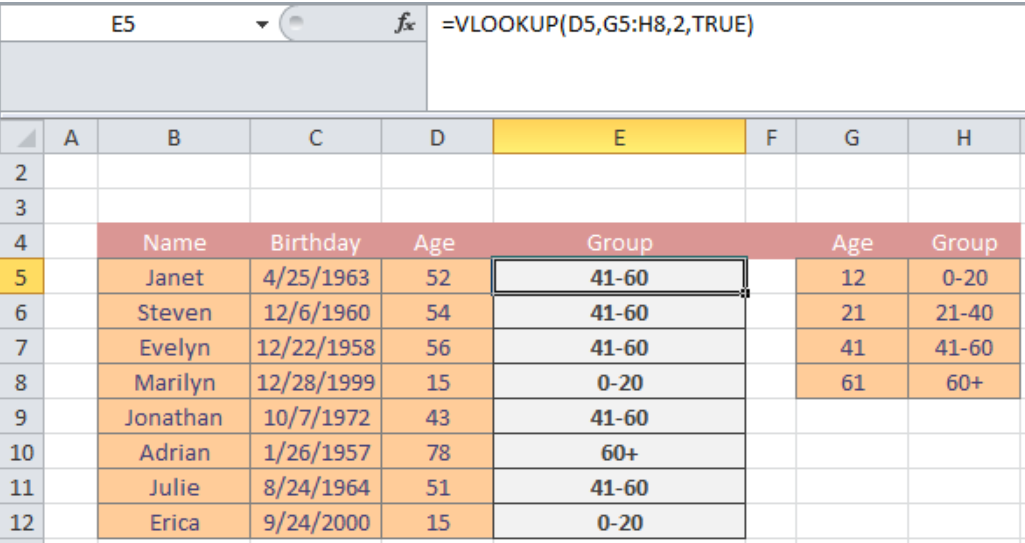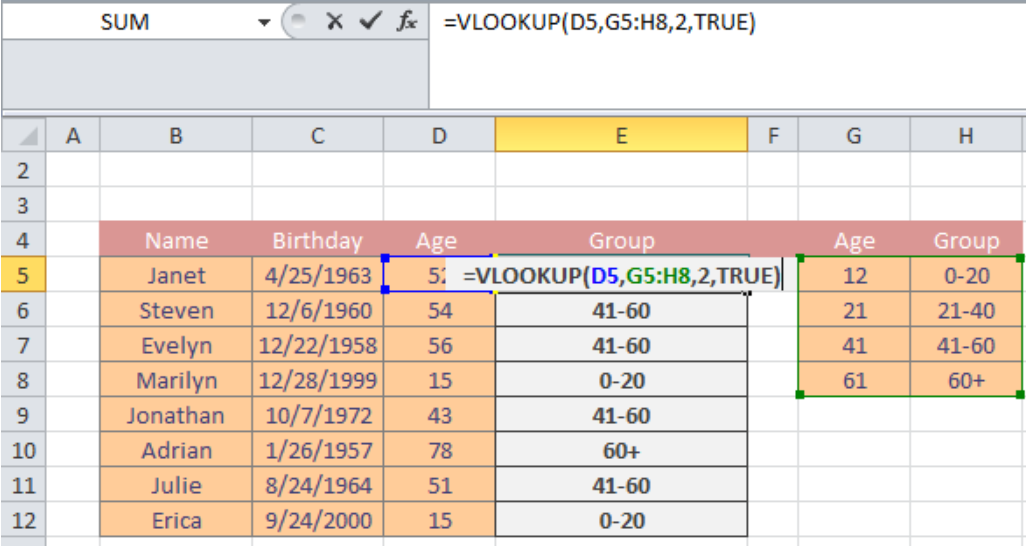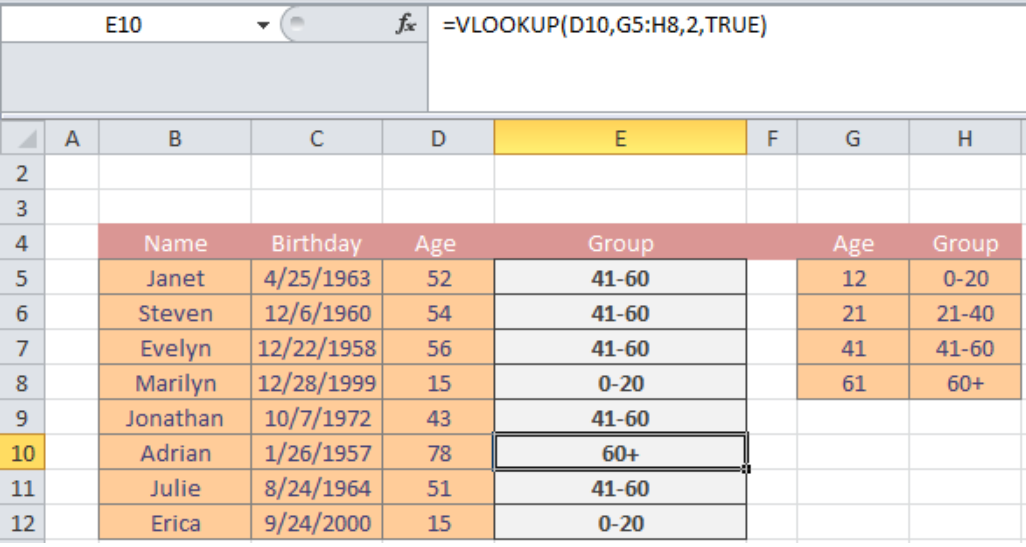Get instant live expert help with Excel or Google Sheets“My Excelchat expert helped me in less than 20 minutes, saving me what would have been 5 hours of work!”

#### Post your problem and you'll get expert help in seconds

Your message must be at least 40 characters
Our professional experts are available now. Your privacy is guaranteed.

# Learn How to Group Numbers with VLOOKUP

When using Excel, we often have a list of groups number, and it is hard to sort by group. Therefore, if your goal is to group by number in Excel, this tutorial will show you how to group numbers with the VLOOKUP function.

## Group numbers in Excel with the VLOOKUP function

You can also categorize the group numbers according to a default function.Figure 1. Example of how to find group numbers with VLOOKUP in Excel

### Formula for VLOOKUP

`=VLOOKUP(value,group_table,column,TRUE)`

### Explanation of formula

If you need to categorize by number then, you can customize the VLOOKUP function for meeting the requirements. The customization enables a user to create arbitrary groups or intervals for grouping numbers with VLOOKUP. For instance, the above-attached screenshot discusses the age vs. age group of people belonging to several demographics. A user is asked to sort out the given ages into group forms.

Here, Column D illustrates ‘age’ which would be taken into factor along with ‘age’ and ‘group’ columns illustrated in Column G and Column H respectively. Next, a user would enter the equation into Column E, where the age group of a corresponding age would be specified—once the user presses ENTER. Let’s have a closer look at how the formula works:

### How does the formula work?

Figure 1 demonstrates the formula bar with the VLOOKUP function. We have used the following formula for sorting group numbers with VLOOKUP custom function:

`=VLOOKUP(D5,G5:H8,2,TRUE)`Figure 2. Example of how to find group numbers with VLOOKUP in Excel

The formula considers the value of D5 as its lookup value. Next, it focuses on G5:H8 as its age_table. Here, 2 indicate that the values are the location in the 2nd column index (Column H). Last but not least, we add TRUE to the equation which serves the purpose of approximate match mode in the equation.

Once we have entered an enclosed the variables and functions—the VLOOKUP function with the scan through the age group, and it would scan the corresponding group name from the 2nd index column. The column can contain customizable text or numeric values as per the preference of the user. Let’s have a look at the following example for understanding the uniqueness and versatility of the formula:Figure 3. Example of how to find group numbers with VLOOKUP in Excel

Still need some help with Excel formatting or have other questions about Excel? Connect with a live Excel expert here for some 1 on 1 help. Your first session is always free.

### Did this post not answer your question? Get a solution from connecting with the expert.Another blog reader asked this question today on Excelchat:## Subscribe to Excelchat.coAnother blog reader asked this question today on Excelchat: